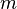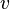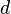# Proof Obligation Names (Rodin User Manual)

Do not edit! This content has been migrated to Subversion.
(Nightly Handbook Build)

Next is a table describing the names of context proof obligations:

 Well-definedness of an Axiom$m$ / WD$m$ is the axiom name Well-definedness of a Derived Axiom$m$ / WD$m$ is the axiom name Derived Axiom$m$ / THM$m$ is the axiom name

Next is a table showing the name of machine proof obligations:

 Well-definedness of an Invariant$v$ / WD$v$ is the invariant name Well-definedness of a Derived Invariant$m$ / WD$m$ is the invariant name Well-definedness of an event Guard$t$ /$d$ / WD$t$ is the event name$d$ is the action name Well-definedness of an event Action$t$ /$d$ / WD$t$ is the event name$d$ is the action name Feasibility of a non-det. event Action$t$ /$d$ / FIS$t$ is the event name$d$ is the action name Derived Invariant$m$ / THM$m$ is the invariant name Invariant Establishment INIT. /$v$ / INV$v$ is the invariant name Invariant Preservation$t$ /$v$ / INV$t$ is the event name$v$ is the invariant name

Next are the proof obligations concerned with machine refinements:

 Guard Strengthening$t$ /$d$ / GRD$t$ is the concrete event name$d$ is the abstract guard name Guard Strengthening (merge)$t$ / MRG$t$ is the concrete event name Action Simulation$t$ /$d$ / SIM$t$ is the concrete event name$d$ is the abstract action name Equality of a preserved Variable$t$ /$v$ / EQL$t$ is the concrete event name$v$ is the preserved variable

Next are the proof obligations concerned with the new events variant:

 Well definedness of Variant VWD Finiteness for a set Variant FIN Natural number for a numeric Variant$t$ / NAT$t$ is the new event name Decreasing of Variant$t$ / VAR$t$ is the new event name

Finally, here are the proof obligations concerned with witnesses:

 Well definedness of Witness$t$ /$p$ / WWD$t$ is the concrete event name$p$ is parameter name or a primed variable name Feasibility of non-det. Witness$t$ /$p$ / WFIS$t$ is the concrete event name$p$ is parameter name or a primed variable name

Remark: At the moment, the deadlock freeness proof obligation generation is missing. If you need it, you can generate it yourself as a derived invariant saying the the disjunction of the abstract guards imply the disjunction of the concrete guards.